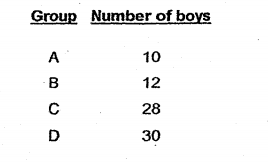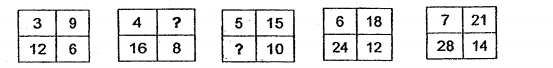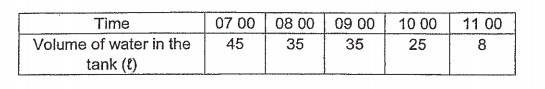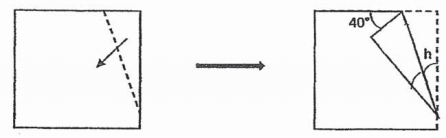# Primary 6 CA1 Practice #2

Time elapsed:
Q1. The price of a concert ticket was \$120. There was an early bird discount of 25% and students were given a further discount of \$12. How much did one early bird discount student's ticket cost?     [1 mark]
Q2. The ratio of the number of pens to pencils to rulers in a stationery shop is 5 : 6 : 3. There are 240 more pencils than rulers. How many pens are there in the shop?     [2 marks]
Q3. Yvette uses syrup and water in the ratio 3 : 5 to make lemon juice. She uses 330 ㎖ of syrup. How much water does she use?     [1 mark]
Q4. 78 of a cake was shared equally among 6 people. What fraction of the cake did each person receive?     [1 mark]
Q5. P and Q are whole numbers. P is the product of all the common factors of 12 and 20. Q is the first common multiple of 3 and 8. What is the value of Q ÷ P?     [2 marks]
Q6. A machine printed 1000 worksheets on the first day and 800 worksheets on the second day. What was the percentage decrease in the number of worksheets printed from the first day to the second day?     [2 marks]
Q7. During a learning journey, the teacher divided all the Primary 6 students into groups of 40. The teacher then wrote down the number of boys in each group. In one of the groups below, the girls made up 30% of the students in the group. Which group was it?     [2 marks]Q8. For every 3 glasses of rose syrup. Kimberly mixes 7 glasses of milk to make some bandung. If the capacity of each glass is 100 ml, how many glasses of milk are needed to make 6 litres of bandung?     [2 marks]
Q9. Olivia and Nathaniel collected some cans during recess for recycling. On Day 1, Olivia collected 12 more cans than Nathaniel. On Day 2, Olivia collected another 36 cans and Nathaniel collected 30 cans. After counting the cans, Olivia collected 611 of the total number of cans. How many cans did Nathaniel collect?     [2 marks]
Q10. Look at the pattern below. Find the sum of the two missing numbers.     [2 marks]Q11. 34 of Alex's stamps is equal to 25 of Samuel's stamps. Find the ratio of the number of Samuel's stamps to the number of Alex's stamps?     [2 marks]
Q12. A tank was filled with 45 ℓ of water at 07 00. Water flowed out from the tank from 07 00 to 08 00 and from 09 00 to 11 00. The table below shows the volume of water in the tank from 07 00 to 11 00. Which one of the graphs below best represents the information in the table?     [2 marks]Q13. Nicole had 45 ℓ of milk. She poured all the milk equally into 8 identical bottles. How many litres of milk did she pour into each bottle?     [1 mark]
Q14. A square piece of paper was folded along the dotted line as shown below. Find ∠h.     [1 mark]Q15. Nicholas bought a pizza. He ate 14 of the pizza and the remaining pizza was shared equally between his 2 friends. What fraction did each of his friend get?     [2 marks]
Q16. Beatrice collected some bookmarks and seashells. The number of bookmarks to the number of seashells was 8 : 5. There were 24 more bookmarks than seashells. What was the total number of bookmarks and seashells in her collection?     [2 marks]
Q17. Express 2 38 as a decimal.     [1 mark]
Q18. Simplify 14a + 8 - 5a - 7.     [1 mark]
Q19. There are 50 pupils in a class. 32 of them are boys. What percentage of the pupils are boys?     [1 mark]
Q20. There were some boys and girls in the school hall. An equal number of boys and girls wear spectacles. 12 of the girls and 47 of the boys do not wear spectacles. What fraction of the children wears spectacles?     [1 mark]